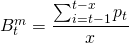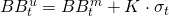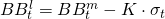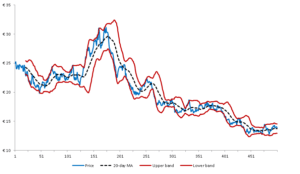# Bollinger bands

Bollinger bands give an indication by the current price of a security lies relatively compared to its past prices. This give you an indication whether a price is more or less expensive compared to the recent past. This is achieved in 2 ways: by measures a simple moving average of past prices and by measuring the price action, volatility of the security.

## Bollinger bands formula

Bollinger bands can be easily calculated using the following procedure. First calculate the middle band. This is simple a simple moving average using a look back period x. A parameter value  of 20 as look back is often found in literature.The upper Bollinger band is simple the sum of the middle band and a multiple, K, of the past standard deviation. A value for K=2 and a look back similar like the calculation of the SMA is used for the standard deviation.Likewise, the lower Bollinger band is calculated by subtracting a multiple of the past standard deviation from the middle band.## Bollinger bands trading strategy

How to use Bollinger bands? Using this technical trading rule, technical analysts could follow various strategies. First one could try to exploit volatility buying (selling) options when volatility is low (high). Second, the following strategy is also often reported by technical traders.  Buy the security when the security is prices at the lower Bollinger band and sell it again when it floats around the middle band. Likewise, selling the security when it is priced at the upper band and buying it back when is floating around the middle band is strategy that can be pursued.

## Summary

Bollinger bands are a popular technical trading rule often used in the financial sector. It measures the relative pricing of a security compared to itself while also indicating the past price action of the security.

### Bollinger bands

Want to have an implementation in Excel? Download the Excel file: bollinger bands